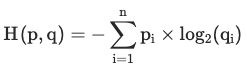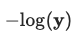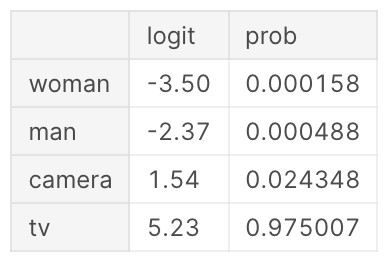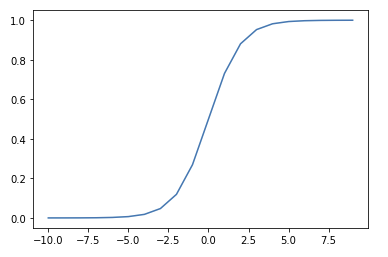• ## Cross-EntropyCross-entropy measures the average number of bits required to identify an event if you had a coding scheme optimised for one probability distribution $q …$

• ## Information EntropyEntropy is a measure of uncertainty of a random variable's possible outcomes.

It's highest when there are many equally likely outcomes. As you introduce more …

• ## Negative Log-LikelihoodNegative log-likelihood is a loss function used in multi-class classification.

Calculated as $-log(\textbf{y})$, where $\mathbf{\text{y …}}$

• ## Softmax Activation FunctionThe Softmax function converts a vector of numbers into a vector of probabilities that sum to 1. It's applied to a model's outputs (or Logits …

• ## Sigmoid Activation FunctionThe Sigmoid function squeezes numbers into a probability-like range between 0 and 1.1 Used in Binary Classification model architectures to compute loss on discrete …

• ## Domain ShiftWhen production data diverges significantly from the training dataset

• ## Rule EnforcerTo prevent cheating, a game needs a rule enforcer.

"if players feel like your game can be cheated, some will try to cheat, but most …

• ## Server Authoritative MultiplayerA typical multiplayer game architecture where the server has authority over the game state. The server keeps track of players' positions, the resources they own …

• ## Out-of-domain dataWhen data is provided to a model that is significantly different from what it was trained on, it's referred to as out-of-domain data.

Howard et …

• ## Metrics Are ProxiesMetrics are usually a proxy for what we really care about